Generating Doubly Stochastic Matrices

Constrained Optimization

Generate random matrix, find closest doubly stochastic in least square sense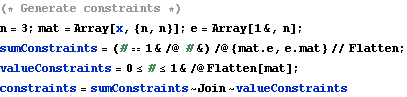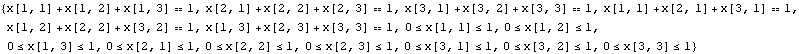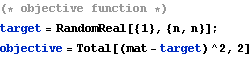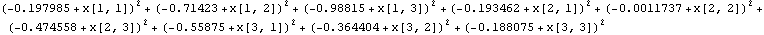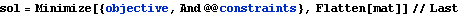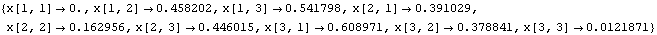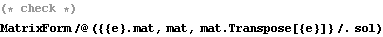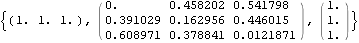Markov Chain sampling

Start with a random permutation matrix, randomly modify 4 entries each time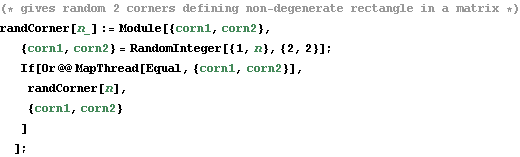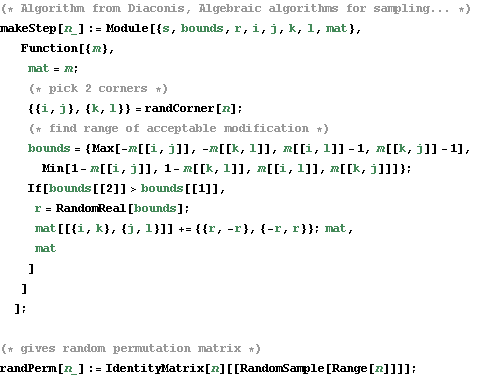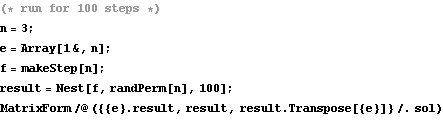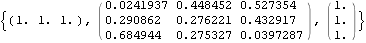Iterative Proportional Fitting (Dr.Teh suggestion)

Normalize rows/columns repeatedly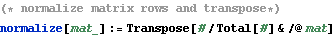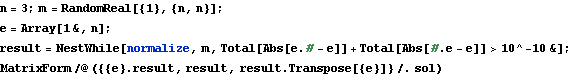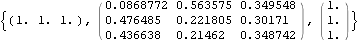How long does it take to converge?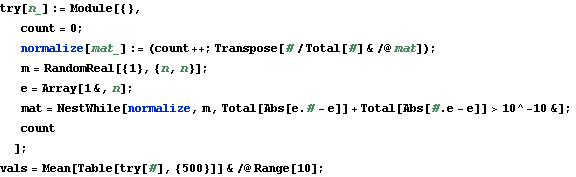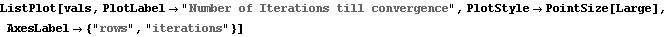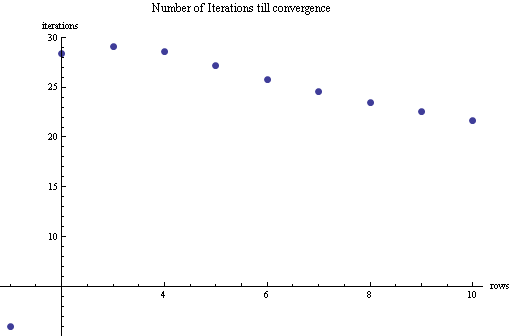Convex Combination of Permutation Matrices (Jeremy's suggestion)

(Birkhoff Theorem, 8.7 .1 from Horn and Johnson)

In:=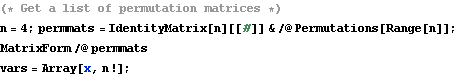Out=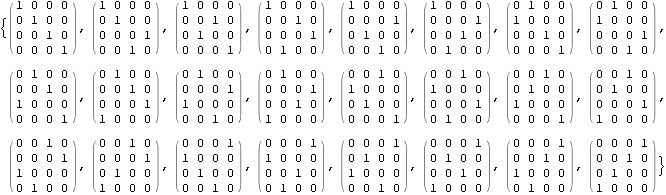In:=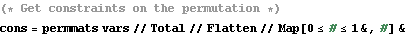Out=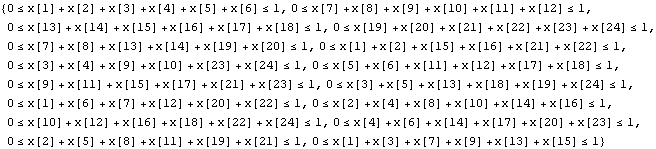In:=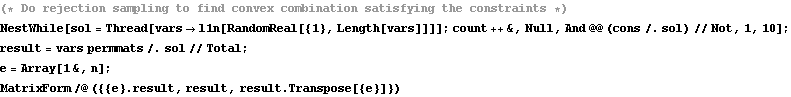Out=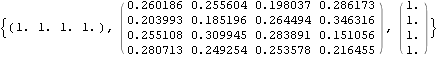Created by Wolfram Mathematica 6.0  (18 December 2007)